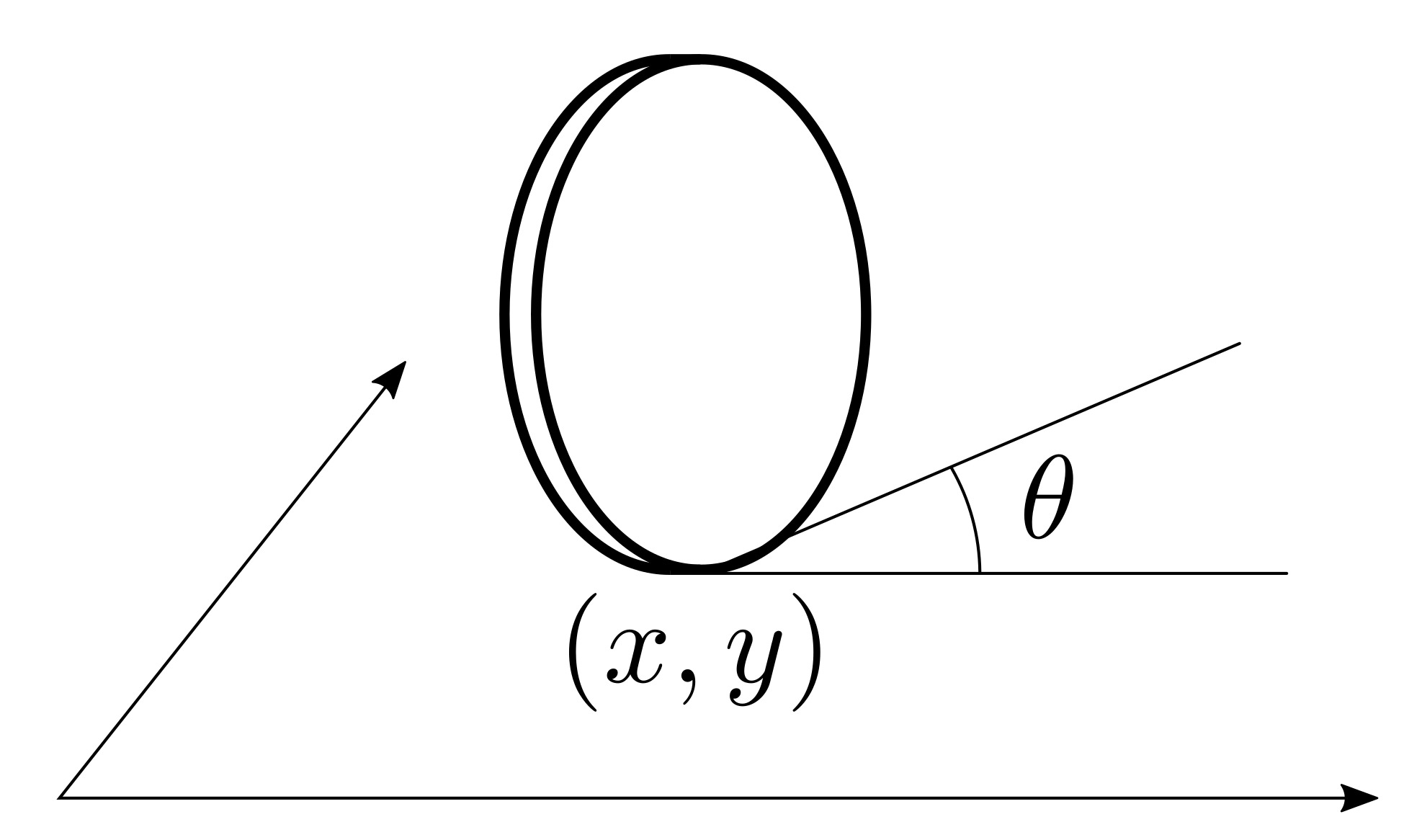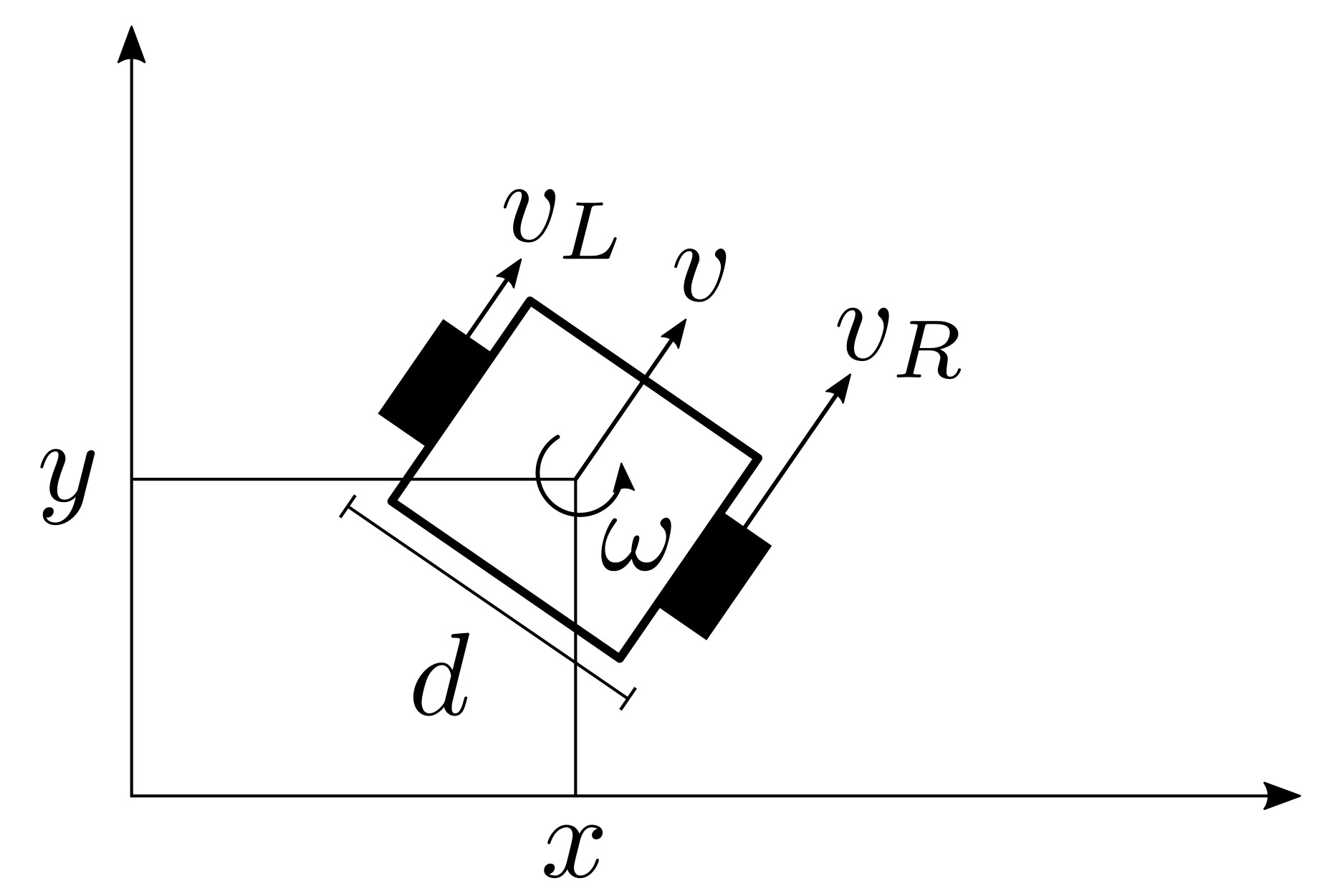# The Hemingwayian unicycle

Published:Unicycles are used to model a large variety of mobile robotic systems: ground, marine, and even aerial robots are very often abstracted using a rolling coin. The dynamic model of the unicycle is given by:

$$\begin{cases} \dot x = v \cos\theta\\ \dot y = v \sin\theta\\ \dot \theta = \omega, \end{cases}$$

where $x$ and $y$ are the coordinates of the position in the plane of the system, $\theta$ is its orientation, and $v$ and $\omega$ are the linear and angular velocity control inputs.

An effective way of designing controllers for unicycle models consists in controlling a point $p$ at a distance $l$ in front of the unicycle depicted in the following figure:In fact, it is easy to see that

$$\dot p = \underbrace{\begin{bmatrix} \cos\theta & -\sin\theta\\ \sin\theta & \cos\theta \end{bmatrix}}_{R(\theta)} \underbrace{\begin{bmatrix} 1 & 0\\ 0 & l \end{bmatrix}}_{L(l)} \begin{bmatrix} v\\ \omega \end{bmatrix}, \tag{1}$$

so, once a controller for $\dot p$ is designed, the inputs $v$ and $\omega$ to the unicycle can be easily computed as

$$\begin{bmatrix} v\\ \omega \end{bmatrix} = L^{-1}(l) R^T(\theta) \dot p. \tag{2}$$As in practice robots are not rolling coins, but rather have wheels, the values $v$ and $\omega$ can be turned into wheel speed in the case of differential-drive robots (shown in the figure above) as follows:

$$\begin{bmatrix} v\\ \omega \end{bmatrix} = \underbrace{\begin{bmatrix} \frac{1}{2} & \frac{1}{2}\\ -\frac{1}{2d} & \frac{1}{2d} \end{bmatrix}}_{D} \begin{bmatrix} v_L\\ v_R \end{bmatrix} \Leftrightarrow \begin{bmatrix} v_L\\ v_R \end{bmatrix} = D^{-1} \begin{bmatrix} v\\ \omega \end{bmatrix} \tag{3}$$

Among the ground mobile robots modeled as unicycles there is the differential-drive BrushBot, which implements a peculiar locomotion strategy that leverages brush elements to turn vibration into locomotion. The locomotion principle is very simple and thus renders the BrushBot robust to interactions with the environment, including external objects or other BrushBots. The motion is achieved by bouncing at a very fast pace on very small pogo sticks (the brushes). And just like with pogo sticks, if you lean forward, you can only move forward. Thus we need a more sophisticated method to properly control these Hemingwayian unicycles.

### A naive approach

To start off, one could consider a version of the unicycle constrained by $v\ge0$—only positive linear velocities allowed, and employ the following controller:

$$\begin{bmatrix} v^\star\\ \omega^\star \end{bmatrix} = \arg\!\min_{v,\omega} \left\{ \left\| \begin{bmatrix} v\\ \omega \end{bmatrix} - L^{-1}(l) R^T(\theta) \dot p \right\|^2 ~\colon~ v\ge0\right\} \tag{4},$$

which is a mouthful for:

1. calculate $v$ and $\omega$ as in (2)
2. let $v^\star=\max \{0,v\}$ and $\omega^\star=\omega$.

The problem with this is that, because of its construction, the differential-drive BrushBot cannot turn in place: $\omega\neq0\implies v\neq 0$. This again is due to the fact that each brush cannot move forward, which is not equivalent to $v\ge0$, but rather to $v_L\ge0$ and $v_R\ge0$ in (3).

### A more sophisticated approach

Enforcing the constraints $v_L\ge0$ and $v_R\ge0$ might lead to the practical impossibility of moving under arbitrary $\dot p$, since, intuitively, not all possible $v$ and $omega$ are simultaneously realizable. To circumvent this issue, we need more degrees of freedom: the idea is that of allowing the distance $l$ to change, and finding its optimal value subject to the constraints that $v_L\ge0$ and $v_R\ge0$.

If $l$ can change over time, (1) becomes

$$\dot p = R(\theta) L(l) D \begin{bmatrix} v_L\\ v_R \end{bmatrix} + \underbrace{\begin{bmatrix} \cos\theta\\ \sin\theta \end{bmatrix}}_{r(\theta)} w,$$

where (3) was substituted in, and $w$ is the rate of change of $l$, which is now an additional control input.

Although $l$ is changing, we might want to drive it—through $w$—to a desired value $\bar l$. Moreover, we would like to keep it positive and less than $\bar l$. The stated conditions lend themselves to be encoded using a control Lyapunov function (CLF) and a control Barrier function (CBF). CLFs and CBFs are control tools which allow us to ensure stability and safety of dynamical systems. In this case the dynamical system is simply

$$\dot l = w,$$

and the stability and safety constraints are

• $l(t) \to \bar l$ as $t\to\infty$
• $l(t) \in [0,\bar l]~\forall t$,

respectively. To this end, we define the following CLF and CBF:

• $V(l) = (l-\bar l)^2$
• $h(l) = l(\bar l-l)$.

Without going too much into the technical details, it suffices to say that using $V$ and $h$ we can turn stability and safety conditions into constraints on the control input $w$, the rate of change of $l$. At this point, we can define the following optimization problem

\begin{aligned} \underset{v_L, v_R, w, \delta}{\mathrm{minimize~}} &\left\| \begin{bmatrix}R(\theta) L(l) D &r(\theta)\end{bmatrix} \begin{bmatrix}v_L\\v_R\\w\end{bmatrix} - \dot p\right\|^2 + \delta^2\\ \mathrm{subject~to~} & v_L\ge0\\ & v_R\ge0\\ & \frac{\partial V}{\partial l} w \le -V(l) + \delta\\ & \frac{\partial h}{\partial l} w \ge -h(l) \end{aligned} \tag{5}

which is a convex quadratic program and, as such, can be solved very efficiently in order to evaluate the values of the control inputs $v_L$ and $v_R$. $\delta$ is just a slack variable which allows us to prioritize the safety constraint (enforced using $h$) over the stability constraint (enforced through $V$), by relaxing the latter.

The results of the application of the described controllers are shown in the following simulation videos.

In all the cases, the differential-drive robot, represented by an arrow, is controlled to drive to the black dot using a simple proportional controller on $p$, the tail of the arrow. Notice how in the first video, the robot moves backwards at the beginning of the trajectory as a result of the mapping (2). As this would be impossible to realize with the Hemingwayian BrushBot, in the second video, the linear velocity is thresholded at 0 according to (4). Nevertheless, in the first part of the trajectory, the robot still turns in place, which, as discussed above, leads to negative wheel velocities. Therefore, in the last video, the controller solution of (5) is used: at the beginning $l$ is reduced (as shown by the length of the arrow and highlighted by the red dot) in order to ensure the $v_L$ and $v_R$ are always positive. The desired value of $l$, $\bar l$ (indicated by the green dot), is tracked as a result of the constraints in (5).

What is Hemingwayian about these unicycles?

“Write drunk, edit sober” is a quote often attributed to Ernest Hemingway. Despite its faithfulness and authenticity, separating writing from editing has been shown to be an effective technique for good writing. As a result, many editors (e.g. ghostwriter, atom) have introduced Hemingway modes, where backspace and delete keys are disabled and it is only possible to add text at the end of a document. This way, you are forced to avoid editing and to only write, to only make forward progress.